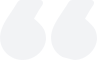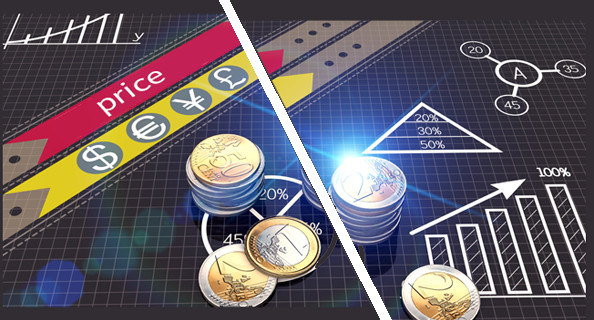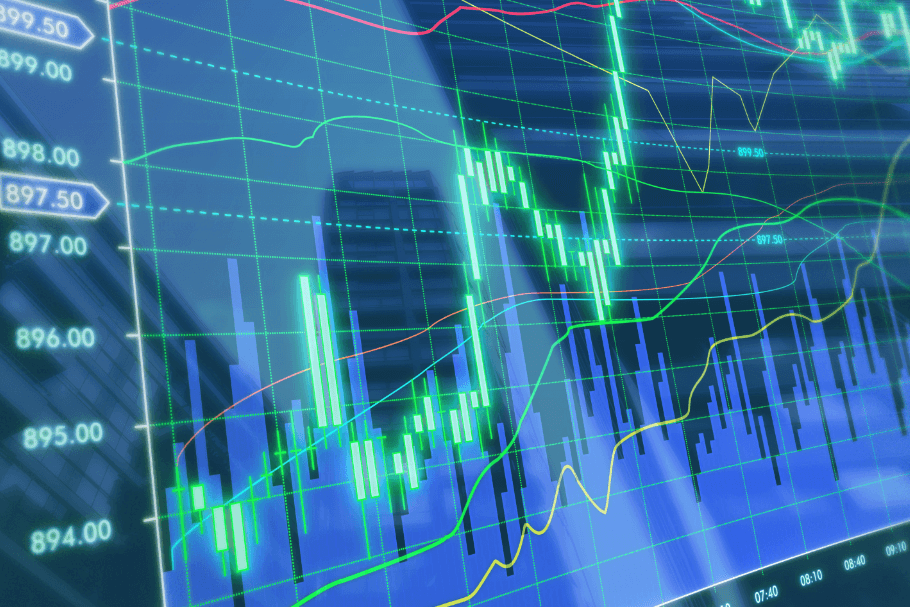# How to calculate the sliding point? How to calculate the sliding point of futures?

2023-06-05Summary:

The calculation of sliding points may vary depending on brokers and trading platforms. Some platforms may not provide real-time sliding point data, but only historical sliding point data. The calculation of futures sliding points may vary depending on factors such as futures varieties, contract specifications, brokers, etc.

How to calculate the sliding point?

Slippage is the difference between the actual transaction price and the expected transaction price, and it is usually calculated using the following formula:

Sliding point=expected transaction price - actual transaction price

Among them, the expected transaction price is the price you see when placing an order, while the actual transaction price is the final execution price of your order. If the actual transaction price is lower than the expected transaction price, the sliding point is negative; If the actual transaction price is higher than the expected transaction price, the sliding point is positive.

For example, if you buy one hand (100000 units) of euros in the euro/dollar currency pair, and the expected transaction price is \$1.2000/euro, but the actual transaction price is \$1.1995/euro, then the sliding point is:

Sliding point=1.2000-1.1995=0.0005 USD/EUR

This means that your actual transaction price is 0.0005 US dollars/euro lower than the expected transaction price, resulting in a sliding point of 0.5 points.

It should be noted that the calculation of sliding points may vary depending on brokers and trading platforms, and some platforms may not provide real-time sliding point data, but only historical sliding point data. Therefore, when choosing trading platforms and brokers, it is necessary to understand their sliding point policies and data provision methods.How to calculate the sliding point of futures?

The calculation method of futures sliding point is similar to that of foreign exchange trading, mainly determined by comparing the difference between the expected transaction price and the actual transaction price.

Specifically, the sliding point of futures can be calculated using the following formula:

Sliding point=transaction price - latest price

Among them, the transaction price is the final execution price of your order, and the latest price is the current latest price in the market. If the transaction price is lower than the latest price, the sliding point is negative; If the transaction price is higher than the latest price, the sliding point is positive.

For example, if you buy one hand (1000 units) of futures on a certain commodity futures contract, and the latest price is 1000 yuan/ton, but your order's final transaction price is 998 yuan/ton, then the sliding point is:

Sliding point=998-1000=-2 yuan/ton

This means that your actual transaction price is 2 yuan/ton lower than the latest price, resulting in a negative sliding point of 2 points.

It should be noted that the calculation of futures sliding points may vary depending on factors such as futures varieties, contract specifications, and brokers. Therefore, when conducting futures trading, it is recommended to understand the sliding point policies of relevant futures contracts and choose reliable brokers for trading.

【 EBC Platform Risk Reminder and Disclaimer 】: There are risks in the market, and investment needs to be cautious. This article does not constitute investment advice.### What is the status of the volume-price relationship?

The volume-price relationship is a key stock market indicator, revealing the correlation between trading volume and stock prices. Analyzing these changes helps investors understand market activity and potential trend reversals.

2023-12-01### What is delisting?

Delisting removes a stock from public trading. It's either voluntary or mandatory, due to violations, financial issues, mergers, etc.

2023-12-01### What does the exchange rate mean?

The exchange rate, reflecting the relative values of two currencies, is influenced by factors like currency supply and demand, balance of payments, economic growth rate, interest rates, monetary policy, and inflation.

2023-11-29